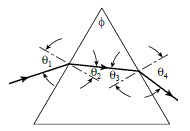# Problem: Light of wavelength 862 nm is incident on the face of a silica prism at an angle of θ  1 = 74.1° (with respect to the normal to the surface). The apex angle of the prism is ∅ = 53.8°. Given: The value of the index of refraction for silica is n = 1.455. Find the angle of refraction at this first surface.

🤓 Based on our data, we think this question is relevant for Professor Yao's class at TEXAS.

###### Problem Details

Light of wavelength 862 nm is incident on the face of a silica prism at an angle of θ  1 = 74.1° (with respect to the normal to the surface). The apex angle of the prism is ∅ = 53.8°. Given: The value of the index of refraction for silica is n = 1.455. Find the angle of refraction at this first surface.